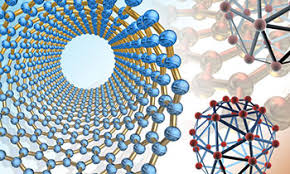## Mechanical & Materials Engineering, Department of## Mechanical & Materials Engineering Faculty Publications

Fall 8-2015

#### Citation

Huijing He, Jiashi Yang and John A. Kosinski. "An estimate of the second-order in-plane acceleration sensitivity of a Y-cut Quartz thickness-shear resonator" IEEE Transactions on Ultrasonics, Ferroelectrics, and Frequency Control Vol. 62 Iss. 8 (2015) p. 1421 - 1428

© 2015 IEEE.

#### Abstract

We perform a theoretical analysis of the second-order in-plane acceleration sensitivity of a Y-cut quartz thick-ness-shear mode resonator. The second-order nonlinear theory of elasticity for anisotropic crystals is used to determine the biasing fields in the resonator under in-plane acceleration. The acceleration-induced frequency shift is determined from a per-turbation analysis based on the plate equations for small-amplitude vibrations superposed on a finite bias. We show that, whereas the first-order acceleration-induced frequency shift is zero for a structurally symmetric resonator under in-plane ac-celeration, the second-order frequency shift is nonzero and is quadratic in the acceleration. As the fourth-order nonlinear elastic constants of quartz have never been measured, we can only estimate the magnitude of the second-order frequency shift. For a particular case of interest, we find ∆ω/ω0 ~ 10^-18, 10^-16 and 10^-14 when the acceleration is 1, 10, and 100 g , respectively.

COinS